Space, shape and measurement: Solve problems by constructing and interpreting trigonometric models

# Unit 2: Problems in two dimensions (2D)

Dylan Busa### Unit outcomes: Unit 2: Problems in two dimensions (2D)

By the end of this unit you will be able to:

• Use a calculator to calculate the value of the three basic trig ratios for different angles.
• Solve problems in two dimensions (2D) using the trigonometric ratios $\scriptsize \cos \theta$, $\scriptsize \sin \theta$, $\scriptsize \tan \theta$.

## What you should know

Before you start this unit, make sure you can:

• Define and use the trigonometric ratios of $\scriptsize \cos \theta$, $\scriptsize \sin \theta$ and $\scriptsize \tan \theta$.
• Calculate the value of expressions containing trigonometric ratios.

To revise these skills, work through Unit 1 of this Subject outcome.

## Introduction

In Unit 1 we defined the three basic trig ratios of sine, cosine and tangent, and we evaluated some expressions that contained these ratios. But we never actually solved any real problems. In this unit, we are going to solve some practical problems with trigonometry. Many of the approaches that we will take are the same as those used every day in the real world.

## Finding the lengths of unknown sides

It’s time to start using trigonometry to solve real problems. One of the most commons types of problems you will encounter is to find the length of an unknown side in a right-angled triangle.

Have a look at the triangle in Figure 1. Because it is a right-angled triangle and because we know the length of two sides, we can easily find the length of the third side $\scriptsize \text{BC}$ by using Pythagoras’ Theorem.

Now look at the triangle in Figure 2. In this case, we only know the length of one of the sides so we cannot use Pythagoras. But we do have another piece of information. We know the size of one of the non-right angles.

With respect to the known angle, which trig ratio combines the length of the side we know ($\scriptsize \text{DE}$) and the length of $\scriptsize x$, the side we are trying to find ($\scriptsize \text{EF}$)?

With respect to $\scriptsize \hat{\text{D}}\,$, we know the length of the adjacent side, and we want to find the length of the opposite side. Therefore, we can use tangent and say that $\scriptsize \tan 34.16{}^\circ =\displaystyle \frac{{\text{opp}}}{{\text{adj}}}=\displaystyle \frac{{\text{EF}}}{{\text{DE}}}=\displaystyle \frac{x}{{5.55}}$. In other words, $\scriptsize \tan 34.16{}^\circ =\displaystyle \frac{x}{{5.55}}$.

Now we have an equation that we can solve.

\scriptsize \begin{align*}\tan 34.16{}^\circ & =\displaystyle \frac{x}{{5.55}}\\\therefore x & =\tan 34.16{}^\circ \times 5.55\end{align*}

But what is the value of $\scriptsize \tan 34.16{}^\circ$? We can use any scientific calculator to find the value of any of the trig ratios for any angle. See the sequence of key presses required for different calculators. You will need to check which sequence your calculator uses.

Casio calculators (direct input): $\scriptsize \boxed{\tan}\to \boxed{34.16}\to\boxed{)}\to\boxed{=}$

Other calculators (indirect input): $\scriptsize \boxed{34.16}\to\boxed{\tan}\to\boxed{=}$

In either case, you should get that $\scriptsize \tan 34.16{}^\circ =0.678579...\approx 0.679$. Note that in this unit we will mostly show the Casio calculator key sequence.

### Note

In most situations the answers you get from your calculator for the value of a trig ratio are non-terminating, non-repeating decimals. For the greatest accuracy, round off only your final answer and round off to three decimal places unless told otherwise.

Now we can solve for $\scriptsize x$ in our original equation.

\scriptsize \begin{align*}\tan 34.16{}^\circ & =\displaystyle \frac{x}{{5.55}}\\\therefore x & =\tan 34.16{}^\circ \times 5.55\\&=3.766\end{align*}

$\scriptsize \boxed{\tan}\to \boxed{34.16}\to\boxed{)}\to\boxed{\times}\to\boxed{5.55}\to\boxed{=}$### Example 2.1

Use your calculator to calculate the following correct to three decimal places:

1. $\scriptsize \cos 58{}^\circ$
2. $\scriptsize 2\times \sin 13.56{}^\circ$
3. $\scriptsize 2{{\sin }^{2}}76.32{}^\circ$
4. $\scriptsize \displaystyle \frac{{\cos 67.3{}^\circ }}{{\sin 41.97{}^\circ }}$

Solutions

1. $\scriptsize \boxed{\cos}\to\boxed{58}\to\boxed{)}\to\boxed{=}\to 0.530$
2. $\scriptsize \boxed{2}\to\boxed{\times}\to\boxed{\sin}\to\boxed{13.56}\to\boxed{)}\to\boxed{=}\to0.469$
3. $\scriptsize \boxed{2}\to\boxed{\times}\to\boxed{\sin}\to\boxed{76.32}\to\boxed{)}\to\boxed{x^2}\to\boxed{=}\to1.888$
4. $\scriptsize \boxed{\cos}\to\boxed{67.3}\to\boxed{)}\to\boxed{\div}\to\boxed{\sin}\to\boxed{41.97}\to\boxed{)}\to\boxed{=}\to0.577$

### Note

When calculating the value of trig ratios, make sure that your calculator is in DEGREE mode. You should see a small D or DEG symbol on screen. If you do not, consult your calculator’s manual for how to change back to DEGREE mode.### Exercise 2.1

1. $\scriptsize \tan 192{}^\circ$
2. $\scriptsize {{\tan }^{2}}12.1{}^\circ$
3. $\scriptsize \sqrt{{\sin 40.2{}^\circ }}$
4. $\scriptsize {{\sin }^{2}}6.67{}^\circ +{{\cos }^{2}}267.7{}^\circ$

The full solutions are at the end of the unit.### Example 2.2

Find the length of $\scriptsize x$ (correct to three decimal places).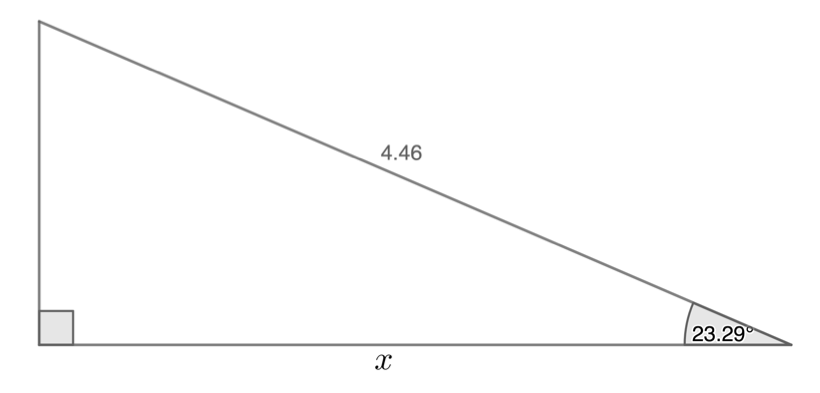Solution

With respect to the angle that we have, we know the hypotenuse and we want the adjacent side. Cosine is the ratio that combines adjacent and hypotenuse.

\scriptsize \begin{align*}\cos 23.29{}^\circ & =\displaystyle \frac{x}{{4.46}}\\\therefore x & =4.46\times \cos 23.29{}^\circ \\ & =4.097\end{align*}

$\scriptsize \boxed{4.46}\to\boxed{\times}\to\boxed{\cos}\to\boxed{23.29}\to\boxed{)}\to\boxed{=}\to4.097$

### Note

If you would like to see some additional simple worked examples watch these two videos:

SOHCAHTOA – Finding Missing Sides PART 1 (Duration: 04.37)SOHCAHTOA – Finding Missing Sides PART 2 (Duration: 02.34)### Exercise 2.2

1. Find the length of $\scriptsize x$ in the following triangles:
1. .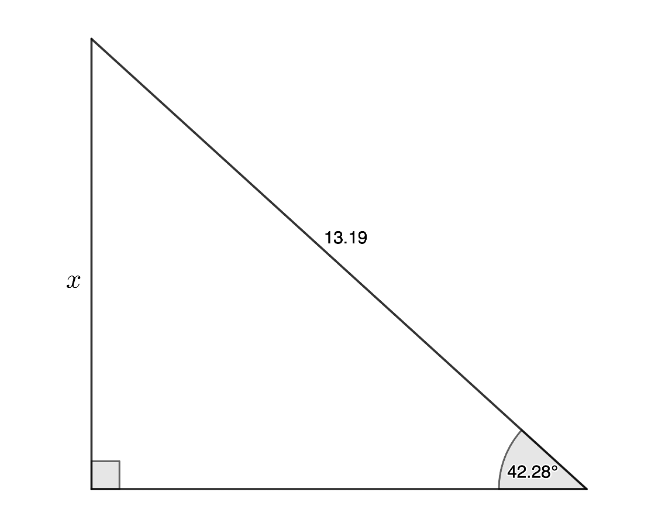2. .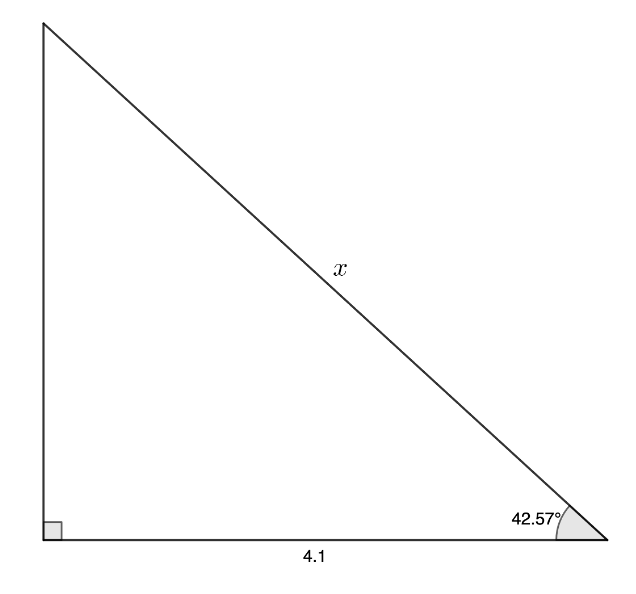2. Calculate $\scriptsize x$ and $\scriptsize y$.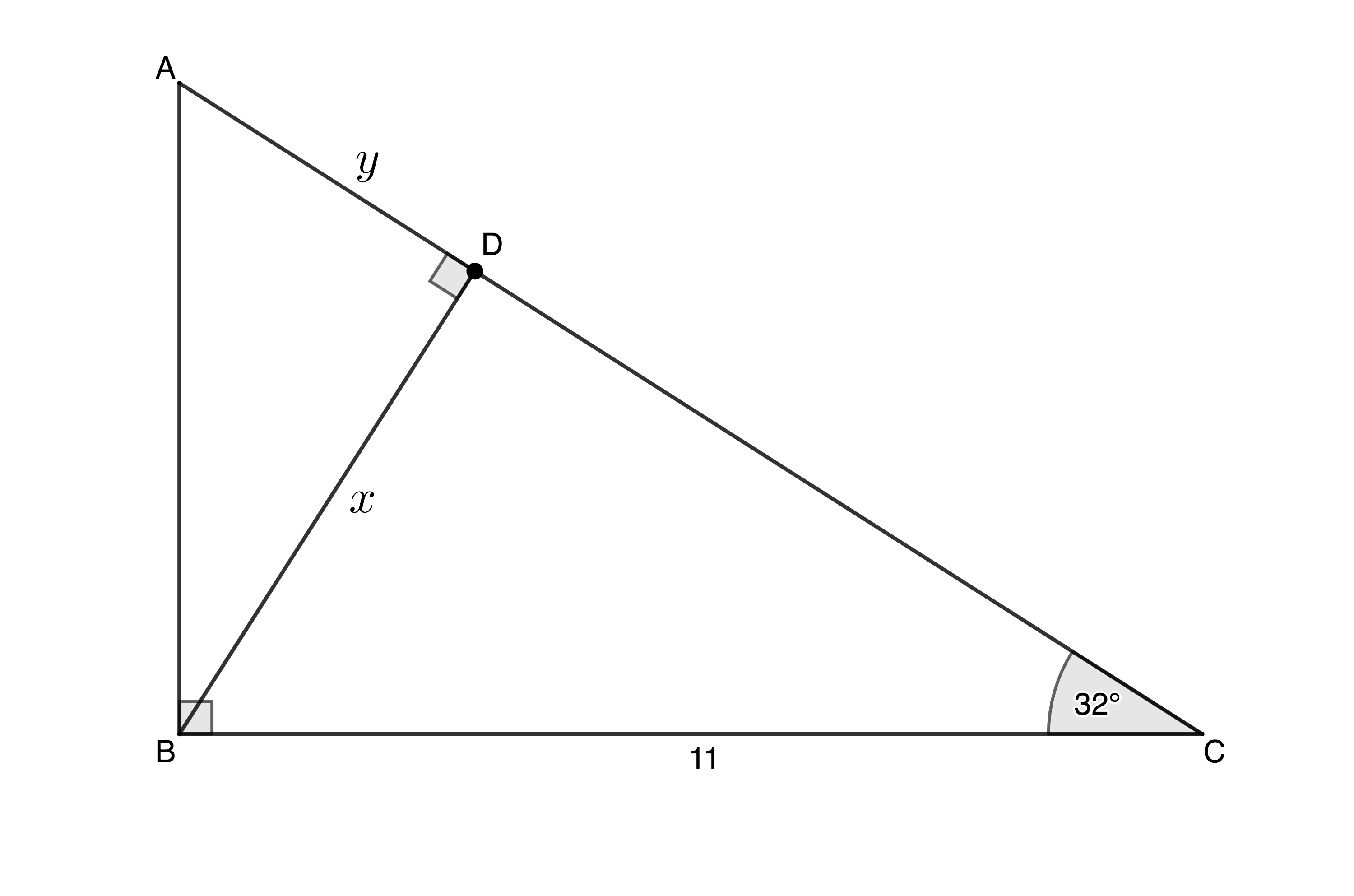The full solutions are at the end of the unit.### Example 2.3

A lighthouse is $\scriptsize 80\ \text{m}$ tall. A ship out at sea measures the angle to the top of the lighthouse as $\scriptsize 13.78{}^\circ$. How far away is the ship from the lighthouse?

Solution

It is often best to draw a sketch of the situation and write down the information given.Relative to the angle that we have, we know the opposite side and we want the adjacent side. Tangent is the ratio that combines opposite and adjacent.

\scriptsize \begin{align*}\tan 13.78{}^\circ & =\displaystyle \frac{{80}}{x}\\\therefore x & =\displaystyle \frac{{80}}{{\tan 13.78{}^\circ }}\\ & =326.193\ \text{m}\end{align*}### Exercise 2.3

Five balls are placed on a pool table as shown in the figure below. The distance between Ball A and Ball B is $\scriptsize 40\ \text{cm}$ while the distance between Ball C and Ball D is $\scriptsize 50\ \text{cm}$. Using the sketch below calculate the distance of Ball A from Ball E ($\scriptsize \text{AE}$) if $\scriptsize \text{AB}$ is parallel to $\scriptsize \text{DE}$ and $\scriptsize \text{C}\overset{\wedge }{\mathop{\text{E}}}\,\text{D}=57{}^\circ$.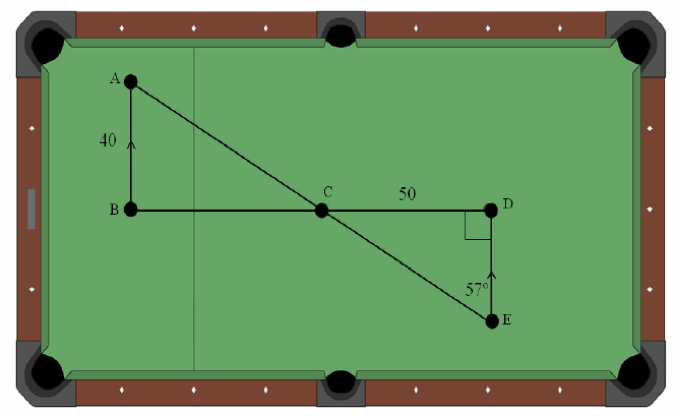The full solutions are at the end of the unit.

## Finding the size of unknown angles

If $\scriptsize \sin 30{}^\circ =\displaystyle \frac{1}{2}$ then it means that if the length of the side opposite an angle and the hypotenuse in a right-angled triangle are in the ratio of 1 : 2, then the angle must be $\scriptsize 30{}^\circ$.

Just like we can use our calculators to find the ratio of the sides when we are given an angle, we can also use them to find the angle if we know the ratio of the sides.

If we want to calculate the angle ($\scriptsize \theta$) that makes the side opposite it and the hypotenuse to be in the ratio $\scriptsize 0.652$, we write that as $\scriptsize \sin \theta =0.652$. Remember that $\scriptsize \sin \theta$ is one entity. We cannot split it. We use a calculator to find the size of $\scriptsize \theta$ as follows:

Casio calculators (direct input): $\scriptsize \boxed{\text{shift/2nd function}}\to\boxed{\sin}\to\boxed{0.652}\to\boxed{)}\to\boxed{=}\to40.693^{\circ}$

Other calculators (indirect input): $\scriptsize \boxed{0.652}\to\boxed{\text{shift/2nd function}}\to\boxed{\sin}\to\boxed{=}\to40.692^{\circ}$

By pressing the SHIFT or 2nd FUNC key, we gain access to the inverse sine or sin-1 function which returns an angle when given a ratio (see Figure 3).

### Note

When calculating the value of an angle, make sure that your calculator is in DEGREE mode. You should see a small D or DEG symbol on screen. If you do not, consult your calculator’s manual for how to change back to DEGREE mode.### Example 2.4

Calculate the value of $\scriptsize \theta$.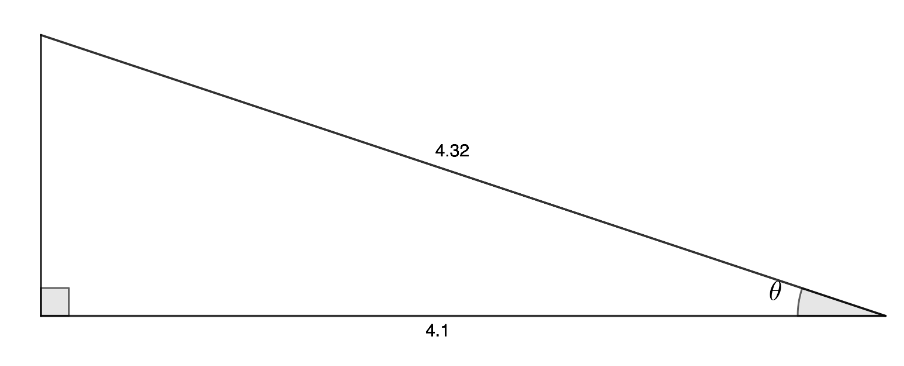Solution

With respect to the angle we want to find, we know the adjacent side and the hypotenuse. Cosine is the ratio that combines adjacent and hypotenuse.

\scriptsize \begin{align*}\cos \theta & =\displaystyle \frac{{\text{adj}}}{{\text{hyp}}}=\displaystyle \frac{{4.1}}{{4.32}}\approx 0.949\\\therefore \theta & =18.364{}^\circ \end{align*}

$\scriptsize \boxed{\text{shift/2nd function}}\to\boxed{\cos}\to\boxed{4.1}\to\boxed{\div}\to\boxed{4.32}\to\boxed{)}\to\boxed{=}\to18.364^{\circ}$

### Note

In most situations the answer you get from your calculator for the value of an angle based on a ratio is a non-terminating, non-repeating decimal. For the greatest accuracy, round off only your final answer and round off to three decimal places unless told otherwise.### Exercise 2.4

1. Find $\scriptsize \theta$ in each case:
1. .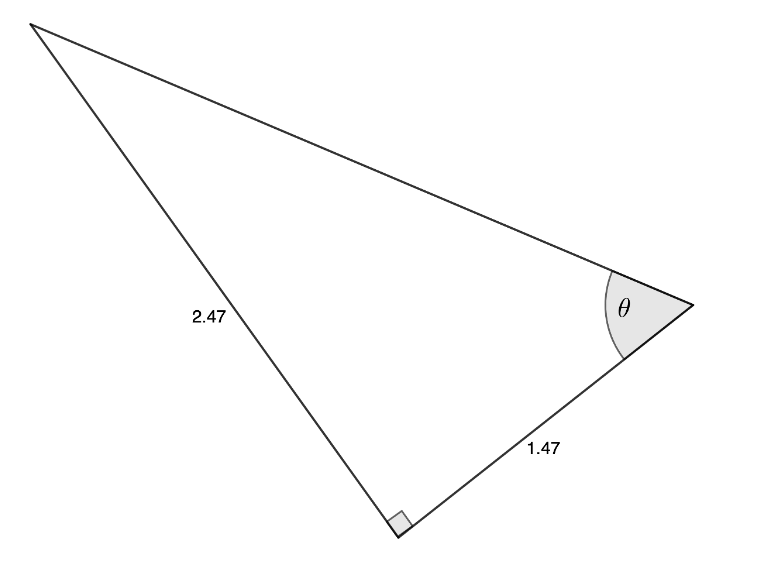2. .2. A river is $\scriptsize 25\ \text{m}$ wide with parallel banks. A swimmer wants to reach a point $\scriptsize 123\ \text{m}$ downstream on the opposite bank. At what angle to the bank of the river does the swimmer need to swim to reach this point?

The full solutions are at the end of the unit.

## Summary

In this unit you have learnt the following:

• How to use a calculator and the trigonometric ratios to calculate the length of a side in a right-angle triangle if you know one angle and the length of one other side.
• How to use a calculator and the trigonometric ratios to calculate the size of an angle in a right-angled triangle if you know the length of two of the sides.
• Solve problems in two dimensions (2D) using the trigonometric ratios $\scriptsize \cos \theta$, $\scriptsize \sin \theta$, $\scriptsize \tan \theta$.

# Unit 2: Assessment

#### Suggested time to complete: 25 minutes

1. Determine $\scriptsize h$ in the following triangle:2. In $\scriptsize \Delta \text{MNP}$, $\scriptsize \text{N}=90{}^\circ$, $\scriptsize \text{MP}=20$ and $\scriptsize \text{P}=40{}^\circ$. Calculate $\scriptsize \text{NP}$ and $\scriptsize \text{MN}$ correct to two decimal places.
3. Determine $\scriptsize x$ and $\scriptsize y$.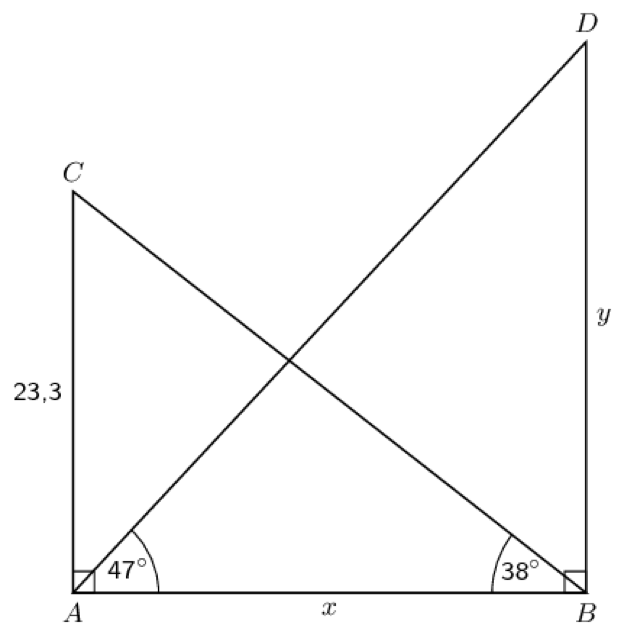4. Light from a laser strikes a flat mirror at an angle of incidence of $\scriptsize \theta$. It reflects off the mirror and strikes a point on a wall $\scriptsize 23\ \text{m}$ away from the laser. If light is reflected at the same angle at which it strikes a mirror and if the mirror and the wall are parallel and a distance of $\scriptsize 120\ \text{cm}$ apart, at what angle does the laser strike the mirror?The full solutions are at the end of the unit.

# Unit 2: Solutions

### Exercise 2.1

1. $\scriptsize \boxed{\tan}\to\boxed{192}\to\boxed{)}\to\boxed{=}\to0.213$
2. $\scriptsize \boxed{\tan}\to\boxed{12.1}\to\boxed{)}\to\boxed{x^2}\to\boxed{=}\to0.046$
3. $\scriptsize \boxed{\sqrt{}}\to\boxed{\sin}\to\boxed{40.2}\to\boxed{)}\to\boxed{=}\to0.803$
4. $\scriptsize \boxed{\sin}\to\boxed{6.67}\to\boxed{)}\to\boxed{x^2}\to\boxed{+}\to\boxed{\cos}\to\boxed{)}\to\boxed{x^2}\to\boxed{=}\to0.015$

Back to Exercise 2.1

### Exercise 2.2

1. .
1. .
\scriptsize \begin{align*}\sin 42.28{}^\circ & =\displaystyle \frac{x}{{13.19}}\\\therefore x & =13.19\sin 42.28{}^\circ & \\ & =8.874\end{align*}
2. .
\scriptsize \begin{align*}\cos 42.57{}^\circ & =\displaystyle \frac{{4.1}}{x}\\\therefore x & =\displaystyle \frac{{4.1}}{{\cos 42.57{}^\circ }}\\ & =5.567\end{align*}
2. .
In $\scriptsize \Delta \text{BCD}$:
\scriptsize \begin{align*}\sin 32{}^\circ & =\displaystyle \frac{x}{{11}}\\\therefore x & =11\sin 32{}^\circ \\ & =5.829\end{align*}In $\scriptsize \Delta \text{ABC}$:
\scriptsize \begin{align*}\text{B}\hat{\text{A}}\text{C} & =180{}^\circ -90{}^\circ -32{}^\circ \ \text{(angles in triangle supplementary)}\\&=58{}^\circ \end{align*}In $\scriptsize \Delta \text{ABD}$:
\scriptsize \begin{align*}\tan 58{}^\circ & =\displaystyle \frac{x}{y}=\displaystyle \frac{{5.829}}{y}\\\therefore y & =\displaystyle \frac{{5.829}}{{\tan 58{}^\circ }}\\ & =3.642\end{align*}

Back to Exercise 2.2

### Exercise 2.3

In $\scriptsize \Delta \text{CDE}$:
\scriptsize \begin{align*}\sin 57{}^\circ &=\displaystyle \frac{{50}}{{\text{CE}}}\\\therefore \text{CE}&=\displaystyle \frac{{50}}{{\sin 57{}^\circ }}\\&=59.618\ \text{cm}\end{align*}
$\scriptsize \text{B}\hat{\text{A}}\text{C}=\text{C}\hat{\text{E}}\text{D}=57{}^\circ \ \text{(alternate angle equal, AB}\parallel \text{DE)}$
<br />
In $\scriptsize \Delta \text{ABC}$:
\scriptsize \begin{align*}\cos 57{}^\circ & =\displaystyle \frac{{40}}{{\text{AC}}}\\\therefore \text{AC} & =\displaystyle \frac{{40}}{{\cos 57{}^\circ }}\\ & =73.443\text{cm}\end{align*}

\scriptsize \begin{align*}\text{AE} & =\text{AC}+\text{CE}\\ & =59.618+73.443\\ & =133.061\ \text{cm}\end{align*}

Back to Exercise 2.3

### Exercise 2.4

1. .
1. .
\scriptsize \begin{align*}\tan \theta & =\displaystyle \frac{{2.47}}{{1.47}}\\\therefore \theta & =59.241{}^\circ \end{align*}
2. .
\scriptsize \begin{align*}\sin \theta & =\displaystyle \frac{{5.66}}{{9.06}}\\\therefore \theta & =38.662{}^\circ \end{align*}
2. .The angle inside the triangle is also $\scriptsize \theta$. Because the banks are parallel, the alternate angles are equal.
\scriptsize \begin{align*}\tan \theta &=\displaystyle \frac{{25}}{{123}}\\\therefore \theta &=11.489{}^\circ \end{align*}

Back to Exercise 2.4

### Unit 2: Assessment

1. .
\scriptsize \begin{align*}\sin 30{}^\circ & =\displaystyle \frac{h}{{20}}\\\therefore h & =20\sin 30{}^\circ \\ & =10\end{align*}
2. .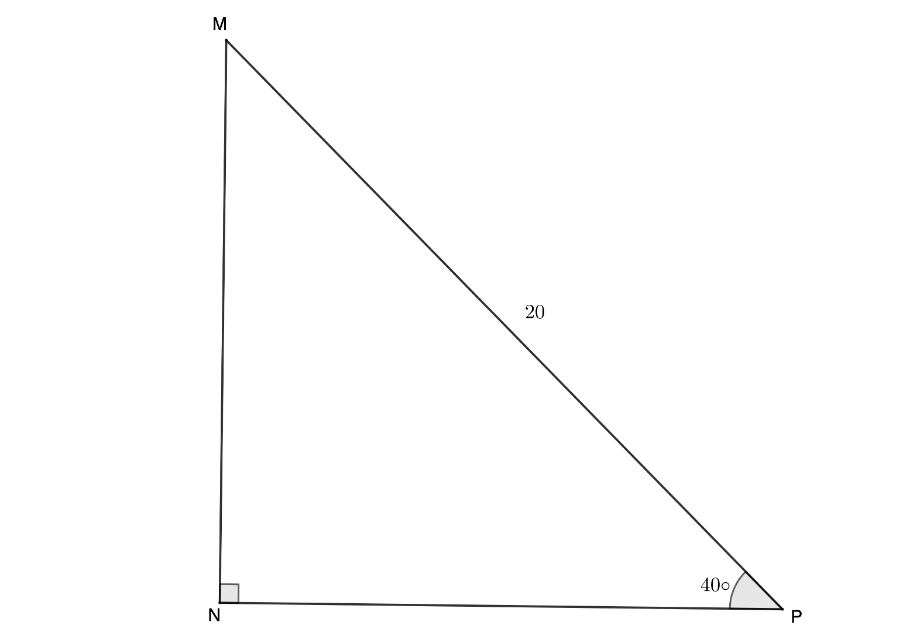\scriptsize \begin{align*}\cos 20{}^\circ & =\displaystyle \frac{{\text{NP}}}{{20}}\\\therefore \text{NP} & =20\cos 20{}^\circ \\ & =18.794\end{align*}
\scriptsize \begin{align*}\sin 20{}^\circ & =\displaystyle \frac{{\text{MN}}}{{20}}\\\therefore \text{MN} & =20\sin 20{}^\circ \\ & =6.840\end{align*}
3. In $\scriptsize \Delta \text{ABC}$:
\scriptsize \begin{align*}\tan 38{}^\circ & =\displaystyle \frac{{23.3}}{x}\\\therefore x & =\displaystyle \frac{{23.3}}{{\tan 38{}^\circ }}\\ & =29.823\end{align*}In $\scriptsize \Delta \text{ABD}$:
\scriptsize \begin{align*}\tan 47{}^\circ & =\displaystyle \frac{y}{x}=\displaystyle \frac{y}{{29.823}}\\\therefore y & =29.823\tan 47{}^\circ \\ & =31.981\end{align*}
4. $\scriptsize \Delta \text{ABD}$ and $\scriptsize \Delta \text{ACD}$ are congruent (same shape and size). Therefore, $\scriptsize \text{BD}=\text{CD}$. But $\scriptsize \text{BC}=23$. Therefore, $\scriptsize \text{BD}=\text{CD}=11.5\ \text{m}$.
In $\scriptsize \Delta \text{ABD}$:
\scriptsize \begin{align*}\tan \theta & =\displaystyle \frac{{11.5}}{{1.2}}\\\therefore \theta & =84.043{}^\circ \end{align*}
Note: $\scriptsize 120\ \text{cm}=1.2\ \text{m}$

Back to Unit 2: Assessment